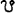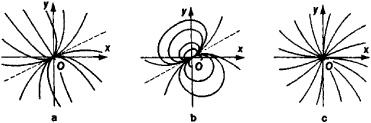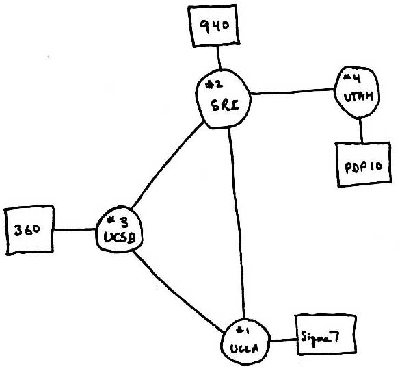node

(redirected from Haygarth nodes)
Also found in: Dictionary, Thesaurus, Medical.
Related to Haygarth nodes: Heberden's nodes

node,

in astronomy, point at which the orbitorbit,
in astronomy, path in space described by a body revolving about a second body where the motion of the orbiting bodies is dominated by their mutual gravitational attraction.
of a body crosses a reference plane. One reference plane that is often used is the plane of the earth's orbit around the sun (eclipticecliptic
, the great circle on the celestial sphere that lies in the plane of the earth's orbit (called the plane of the ecliptic). Because of the earth's yearly revolution around the sun, the sun appears to move in an annual journey through the heavens with the ecliptic as its
). Since the moon's orbit has an inclinationinclination,
in astronomy, the angle of intersection between two planes, one of which is an orbital plane. The inclination of the plane of the moon's orbit is 5°9' with respect to the plane of the ecliptic (the plane of the earth's orbit around the sun).
of 5°9' to the plane of the ecliptic, there are two nodes in the moon's orbit around the earth; the point where the moon in its orbit crosses from south of the ecliptic plane to north of it is called the ascending node, and the point where it crosses from north to south is called the descending node. A line connecting two nodes is called a line of nodes. The lunar nodes are the points where the moon's line of nodes, when extended, strike the celestial spherecelestial sphere,
imaginary sphere of infinite radius with the earth at its center. It is used for describing the positions and motions of stars and other objects. For these purposes, any astronomical object can be thought of as being located at the point where the line of sight
. The lunar nodes regress (move westward along the ecliptic) due to perturbationsperturbation
, in astronomy and physics, small force or other influence that modifies the otherwise simple motion of some object. The term is also used for the effect produced by the perturbation, e.g., a change in the object's energy or path of motion.
from the other bodies in the solar system, e.g., the sun and planets. Another reference plane that can be used to define nodes is the plane of the earth's equator, which is also the plane of the celestial equator (see equatorial coordinate systemequatorial coordinate system,
the most commonly used astronomical coordinate system for indicating the positions of stars or other celestial objects on the celestial sphere. The celestial sphere is an imaginary sphere with the observer at its center.
). There are two nodes in the sun's apparent orbit around the earth. The ascending node, when the sun appears to cross the celestial equator from south to north, is the vernal equinoxequinox
, either of two points on the celestial sphere where the ecliptic and the celestial equator intersect. The vernal equinox, also known as "the first point of Aries," is the point at which the sun appears to cross the celestial equator from south to north.
; the descending node is the autumnal equinox. Perturbations like those that cause regression of the lunar nodes cause the precession of the equinoxesprecession of the equinoxes,
westward motion of the equinoxes along the ecliptic. This motion was first noted by Hipparchus c.120 B.C. The precession is due to the gravitational attraction of the moon and sun on the equatorial bulge of the earth, which causes the earth's axis to
.

Node

in astronomy, one of the two diametrically opposite points of the celestial sphere at which the plane of the orbit of some celestial body intersects a reference coordinate plane that is either the plane of the ecliptic or of the equator. The points where the orbit intersects the reference plane are sometimes also called nodes. A distinction is made between the ascending node (astronomical symbol), where the celestial body crosses from the southern to the northern side of the reference plane, and the descending node (symbol), where the body crosses the reference plane in the opposite direction.

For planets, comets, and the moon the ecliptic serves as the reference plane. In this case, the position of the nodes on the celestial sphere is defined by their longitudes. When the orbits of artificial earth satellites are determined, the plane of the equator serves as the reference plane, and the positions of the nodes are given by the right ascensions of the nodes. The longitude of the ascending node and the right ascension of the ascending node are orbital elements.

For binary stars the points where the orbits intersect the plane perpendicular to the line of sight are called nodes.

Node

in botany, the part of a shoot axis on which the leaf, the bud, and, sometimes, the adventitious roots form.

Nodes are separated by internodes. On the basis of the distance between nodes, shoots are distinguished as being long or short. Nodes form on the growing point of a shoot when the leaf rudiments first appear. At the node the procambial bundles of the developing leaf become embedded in the axis of the shoot. From these bundles and from the bundles of previously laid down leaves the common bundles of the shoot axis are formed. The common bundles constitute the entire conducting system of the axis. The anatomical structure of nodes varies, depending on the leaf arrangement, the number of procambial bundles in the leaf, and the way the bundles enter the node. Bundles of the axillary buds (branches), which form branch traces, join the conducting system of the shoot axis at the node. The structure of the shoot nodes is a taxonomic character for many plant species.

Node

in mathematics, a type of singular, or critical, point of a differential equation (seeSINGULAR POINT). All integral curves passing through points in a sufficiently small neighborhood of a node enter the node.

In Russian mathematical literature, three different types of such nodes are distinguished: ordinary (obychnyi), degenerate (vyrozhdennyi), and special (osobyi). An ordinary node is a critical point such that all but one of the integral curves passing through the point are tangent to the same line (Figure l, a). In the case of a degenerate node, all the integral curves passing through the node are tangent to the same line (Figure l, b). In the case of a special, or dicritical (dikriticheskii), node, integral curves enter the origin from every direction (Figure l, c). In English, the first two cases are sometimes called improper nodes, and the third case is sometimes referred to as a proper node.Figure 1

The term “node” is also applied to one type of singular point of a curve.

The Russian word for node—uzel—also has the meaning of knot. In topology a knot is, in the simplest case, a continuous closed space curve that does not intersect itself.

Node

in physics, a point or surface in a standing-wave system at which the kinetic or potential energy of the wave is zero. In a stretched flexible string exhibiting sinusoidal free vibrations, tension nodes alternate with displacement nodes. In a standing sound wave, pressure nodes alternate with velocity nodes. In both cases, positions of zero potential energy alternate with positions of zero kinetic energy. Potential energy nodes coincide with kinetic energy antinodes, or loops, and kinetic energy nodes coincide with potential energy antinodes. In electromagnetic standing waves, nodes of the electric and magnetic fields are positions of zero electric and magnetic field strength, respectively; nodes of the electric field are antinodes of the magnetic field, and. vice versa.

node

[nōd]
(anatomy)
A knob or protuberance.
A small, rounded mass of tissue, such as a lymph node.
(astronomy)
One of two points at which the orbit of a planet, planetoid, or comet crosses the plane of the ecliptic.
One of two points at which a satellite crosses the equatorial plane of its primary.
(botany)
A site on a plant stem at which leaves and axillary buds arise.
(electricity)
(electronics)
A junction point within a network.
(geology)
That point along a fault at which the direction of apparent displacement changes.
(industrial engineering)
On a graphic presentation of a project, a symbol placed at the intersection of arrows that represent activities to identify the completion or start of an activity.
(mathematics)
(astronomy)
(neuroscience)
A point of constriction along a nerve.
(physics)
A point, line, or surface in a standing-wave system where some characteristic of the wave has essentially zero amplitude.

node

1. In electric wiring, a junction point at which several distribution or wiring conductors come together.

panel point, node

A point where members of a truss intersect.

node

1. the point on a plant stem from which the leaves or lateral branches grow
2. Physics a point at which the amplitude of one of the two kinds of displacement in a standing wave has zero or minimum value. Generally the other kind of displacement has its maximum value at this point
3. Maths a point at which two branches of a curve intersect, each branch having a distinct tangent
4. Maths Linguistics one of the objects of which a graph or a tree consists; vertex
5. Astronomy either of the two points at which the orbit of a body intersects the plane of the ecliptic. When the body moves from the south to the north side of the ecliptic it passes the ascending node and from the north to the south side it passes the descending node
6. Anatomy
a. any natural bulge or swelling of a structure or part, such as those that occur along the course of a lymphatic vessel (lymph node)
b. a finger joint or knuckle
7. Computing an interconnection point on a computer network

node

(1)
A point or vertex in a graph.

(2)

node

(3)
A hypertext document.

node

(1) See Node.js.

(2) In a network, a node is a junction or connection point. Every terminal, computer, hub, switch and router is a node. Devices that hold and serve data are "hosts." See host, hub, switch, router and terminal.

(3) In database management, a node is an item of data that can be accessed by two or more routes. See DBMS.

(4) In the Document Object Model (DOM), which exposes HTML and XML content to an application or script, every element, every attribute of that element, and each piece of textual content for every attribute is considered a node. See DOM.

(5) In computer graphics, a node is an endpoint of a graphical element. See graphics.

(6) In multiprocessing systems, a node can be a single processor or system. In MPP, it is one processor. In SMP, it is one computer system with two or more processors and shared memory. See SMP and MPP.

The First Four Nodes of the InternetScrawled on this paper in 1969 were the first four nodes of the ARPANET network, which later became the Internet. (Image courtesy of The Computer History Museum, www.computerhistory.org)
Site: Follow: Share:
Open / Close